Question

A 1.80-cm-high insect is 1.45 m from a 139-mm-focal-length lens. Where is the image? Follow the sign conventions.How high is the image? Follow the sign conventions.What type is the image?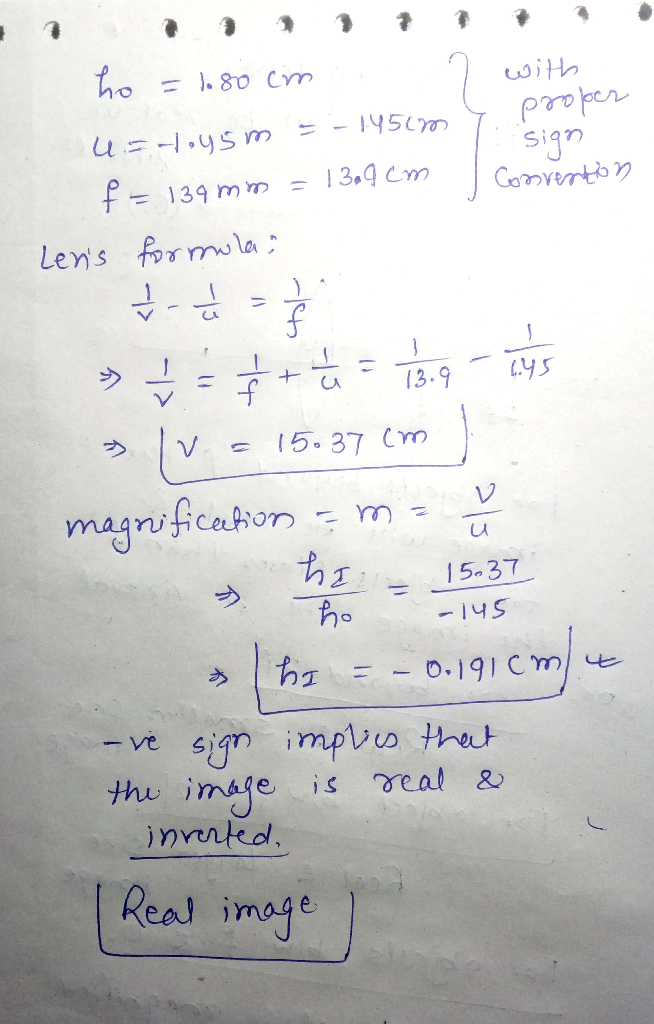#### Earn Coins

Coins can be redeemed for fabulous gifts.

Similar Homework Help Questions
• ### A 2.70-cm-high insect is 1.45 m from a 131-mm-focal-length lens. Where is the image? Follow the sign conventions.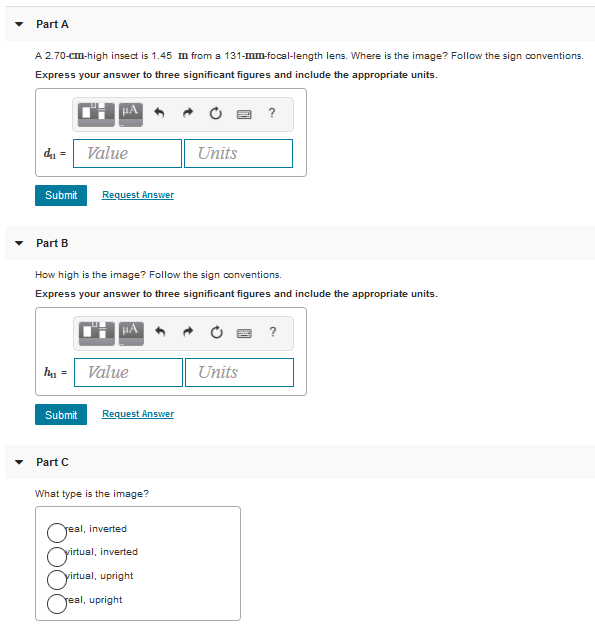A) A 2.70-cm-high insect is 1.45 m from a 131-mm-focal-length lens. Where is the image? Follow the sign conventions.B) How high is the image? Follow the sign conventions.C) What type is the image?

• ### A 2.11 cm high insect is 1.31 m from a -125 mm focal-length lens

A 2.11 cm high insect is 1.31 m from a -125 mm focal-length lens. Where is the image? Distance of the image is ? How high is it? What type is it?

• ### (a) A 2.20 cm high insect is 1.24 m from a 135 mm focal-length lens

(a) A 2.20 cm high insect is 1.24 m from a 135 mm focal-length lens. What is the image distance? mm How high is the image? cm What type is it? (Select all that apply.) The image is upright. The image is inverted. The image is behind the lens. The image is in front of the lens. The image is virtual. The image is real. (b) What if f = -135 mm? What is the image distance? mm How high...

• ### A converging lens with a focal length of 8.00 cm forms an image of a 5.60-mm-tall...

A converging lens with a focal length of 8.00 cm forms an image of a 5.60-mm-tall real object that is to the left of the lens. The image is 1.40 cm tall and erect. a) Where is the object located? Follow the sign rules. (express answer with appropriate units) b) Where is the image located? Follow the sign rules. (express answer with appropriate units)

• ### A small insect is placed 5.50 cm from a 6.00-cm-focal-length lens. a) Calculate the position of...

A small insect is placed 5.50 cm from a 6.00-cm-focal-length lens. a) Calculate the position of the image. Follow the sign conventions. Express your answer using two significant figures and include appropriate units. b) Calculate the angular magnification. Express your answer using two significant figures.

• ### A concave lens has a focal length of -36 cm. Find the image distance and magnification...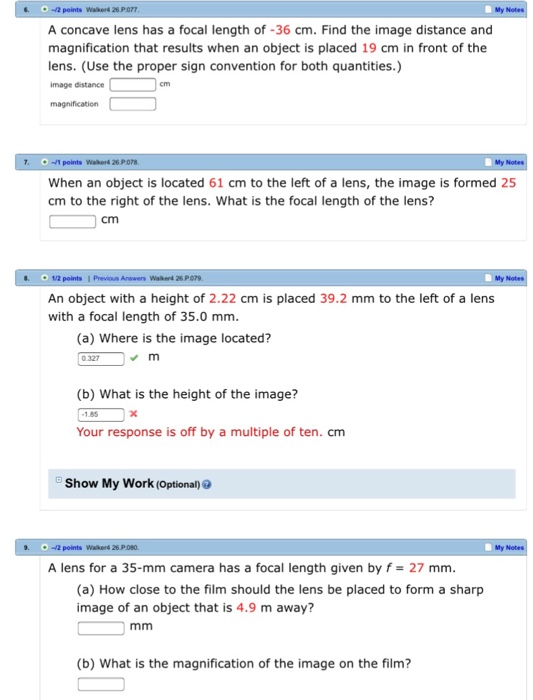A concave lens has a focal length of -36 cm. Find the image distance and magnification that results when an object is placed 19 cm in front of the lens. (Use the proper sign convention for both quantities.) When an object is located 61 cm to the left of a lens, the image is formed 25 cm to the right of the lens. What is the focal length of the lens? An object with a height of 2.22 cm is...

• ### A small insect is placed 6.50 cm from a 7.00-cm-focal-length lens. Calculate the angular magnification. Express...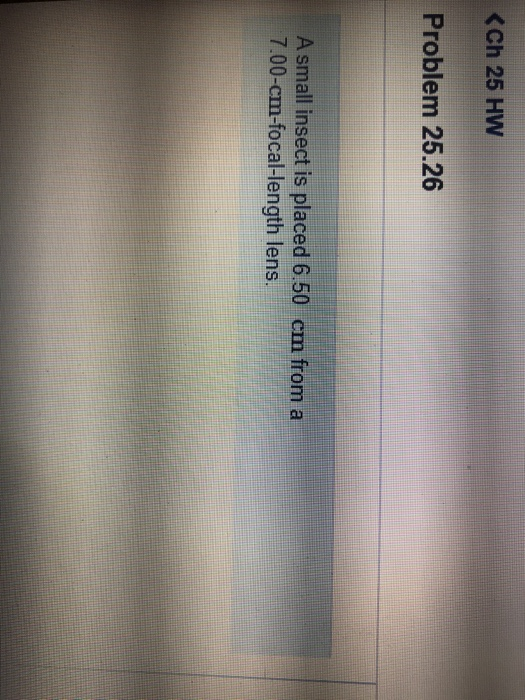A small insect is placed 6.50 cm from a 7.00-cm-focal-length lens. Calculate the angular magnification. Express your answer using two significant figures. calculate the angular magnification, the distance of the image is -91cm <Ch 25 HW Problem 25.26 A small insect is placed 6.50 cm from a 7.00-cm-focal-length lens.

• ### A certain lens focuses light from an object 1.25 m away as an image 41.8 cm on the other side of the lens. What is its focal length?A certain lens focuses light from an object 1.25 m away as an image 41.8 cm on the other side of the lens.1) What is its focal length? Follow the sign conventions.

• ### An image is 4.0 mm in front of a converging lens with focal length 5.0 mm. Where is the object?

An image is 4.0 mm in front of a converging lens with focal length 5.0 mm. Where is the object?A. 9.0 mm in front of the lensB. 2.2 mm behind the lensC. 2.2 mm in front of the lensD. 20 mm in front of the lensE. 9.0 cm behind the lens

• ### A converging lens with a focal length of 12 cm produces a 3-cm high virtual image...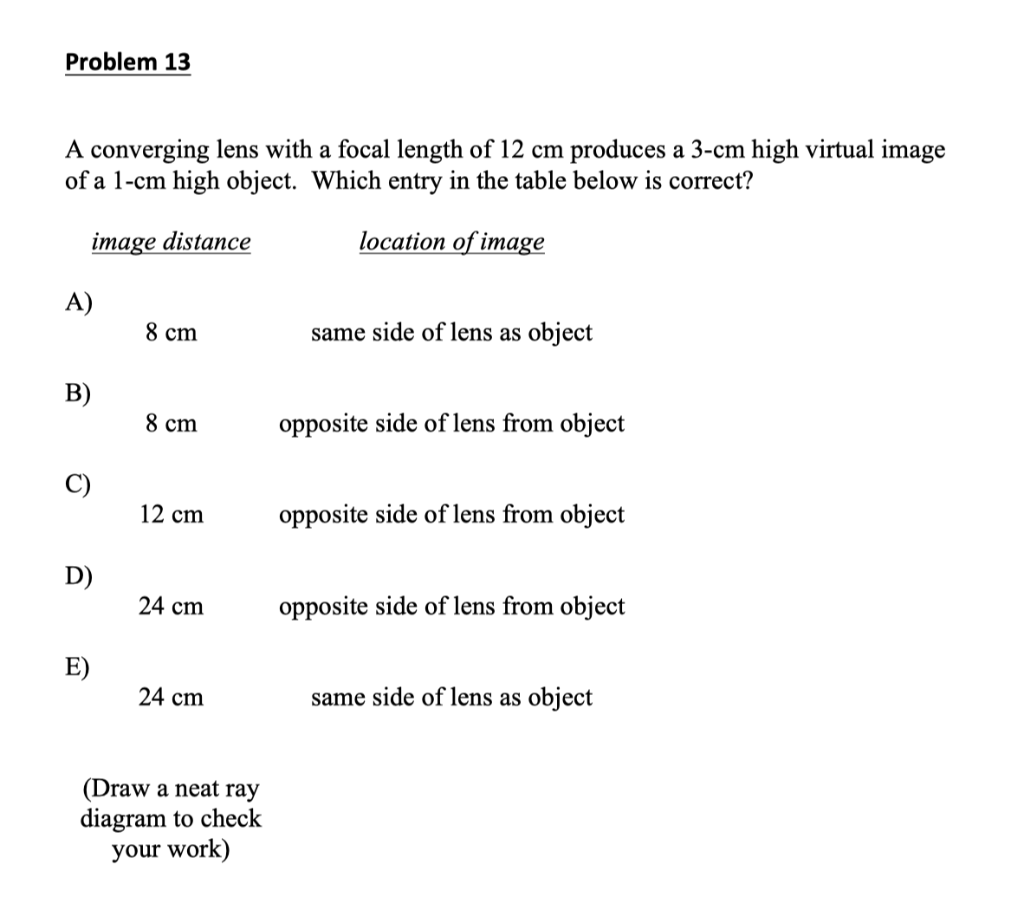A converging lens with a focal length of 12 cm produces a 3-cm high virtual image of a 1-cm high object. Which entry in the table below is correct? Draw a neat ray diagram. PLEASE REMEMBER TO DRAW THE RAY DIAGRAM. Problem 13 A converging lens with a focal length of 12 cm produces a 3-cm high virtual image of a 1-cm high object. Which entry in the table below is correct? image distance location of image A) 8 cm...